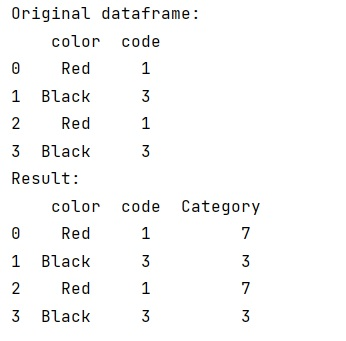# Conditionally fill column values based on another columns value in Pandas

Learn, how to conditionally fill column values based on another columns value in Pandas?
Submitted by Pranit Sharma, on February 07, 2023

NumPy is an abbreviated form of Numerical Python. It is used for different types of scientific operations in python. Numpy is a vast library in python which is used for almost every kind of scientific or mathematical operation. It is itself an array which is a collection of various methods and functions for processing the arrays.

Pandas is a special tool that allows us to perform complex manipulations of data effectively and efficiently. Inside pandas, we mostly deal with a dataset in the form of DataFrame. DataFrames are 2-dimensional data structures in pandas. DataFrames consists of rows, columns, and data.

## Problem statement

Suppose that we are given a dataframe that contains a column of different colour names and another column contains the colour code and we need to add an extra column that contains values depending upon the other column values. Suppose this new column is the category and it is some calculated values if colour is RED.

## Conditionally fill column values based on another columns value

For this purpose, we will simply access the colour column by using the np.where() method with dataframe column and apply the necessary condition.

Let us understand with the help of an example,

## Python program for conditionally fill column values based on another columns value

```# Import numpy
import numpy as np

# Importing pandas package
import pandas as pd

# Creating dictionary
d = {
'color':['Red','Black','Red','Black'],
'code':[1,3,1,3]
}

# Creating dataframe
df = pd.DataFrame(d)

# Display original dataframe
print("Original dataframe:\n",df)

# Adding new column based on other two columns
df['Category'] = np.where(df['color'] == 'Red', df['code'] * 7, df['code'])

# Display result
print("Result:\n",df,"\n")
```

### Output

The output of the above program is: